TitleCollege Algebra Tutorial 3: Scientific Notation

WTAMU > Virtual Math Lab > College AlgebraLearning Objectives

 After completing this tutorial, you should be able to: Write a number in scientific notation. Write a number in decimal notation without exponents.Introduction

 This tutorial takes a look at the basic definition of scientific notation, an application that involves writing the number using an exponent on 10.  Since part of a number that is written in scientific notation is 10 raised to a power, when we multiply or divide these types of numbers we need to remember some of our exponent rules.  If you need to review your exponent rules, feel free to go to Tutorial 2:  Integer Exponents.  Like it or not, the best way to master these problems is to work through them.  So I guess we better get to it.Tutorial

 Scientific Notation A positive number is written in scientific notation  if it is written in the form: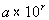where 1 < a < 10 and r is an integer power of 10.

 Writing a Number in Scientific Notation

 Step 1:  Move the decimal point so that you have a number that is between 1 and 10.

 In other words, you will put your decimal after the first non zero number.

 Step 2:   Count the number of decimal places moved in Step 1 .

 If the decimal point was moved to the left, the count is positive. If the decimal point is moved to the right, the count is negative.

 Step 3:   Write as a product of the number (found in Step 1) and 10 raised to the power of the count (found in Step 2).Example 1:    Write the number in scientific notation:   734,000,000,000.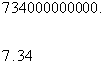*Decimal is at the end of the number   *Move decimal to create a number between 1 and 10

 How many decimal places did we end up moving? We started at the end of the number 734,000,000,000 and moved it between the 7 and 3.  That looks like a move of 11 places. What direction did it move? Looks like we moved it to the left. So, our count is +11.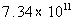Note how the number we started with is a bigger number than the one we are multiplying by in the scientific notation.  When that is the case, we will end up with a positive exponent.Example 2:    Write the number in scientific notation:   .000015.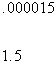*Decimal is at the beginning of the number   *Move decimal to create a number between 1 and 10

 How many decimal places did we end up moving? We started at the beginning of the number .000015  and moved it between the 1 and 5.  That looks like a move of 5 places. What direction did it move? Looks like we moved it to the right. So, our count is - 5.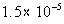Note how the number we started with is a smaller number than the one we are multiplying by in the scientific notation.  When that is the case we will end up with a negative exponent.

 Write a Scientific Number in Standard Form

Basically, you just multiply the first number times the power of 10.

 Whenever you multiply by a power of 10, in essence what you are doing is moving your decimal place. If the power on 10 is positive, you move the decimal place that many units to the right. If the power on 10 is negative, you move the decimal place that many units to the left. Make sure you add in any zeros that are neededExample 3:  Write the number in decimal notation without exponents: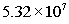.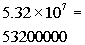*Move the decimal 7 to the rightExample 4:  Write the number in decimal notation without exponents: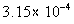.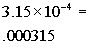*Move the decimal 4 to the leftExample 5:  Perform the indicated operation.  Express the answer in decimal form.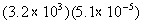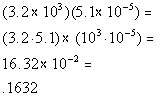*When mult. like bases you add your exponents: 3 + (-5) = -2 *Move the decimal 2 to the leftExample 6:  Perform the indicated operation.  Express the answer in decimal form.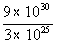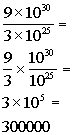*Move the decimal 5 to the rightPractice Problems

 These are practice problems to help bring you to the next level.  It will allow you to check and see if you have an understanding of these types of problems. Math works just like anything else, if you want to get good at it, then you need to practice it.  Even the best athletes and musicians had help along the way and lots of practice, practice, practice, to get good at their sport or instrument.  In fact there is no such thing as too much practice. To get the most out of these, you should work the problem out on your own and then check your answer by clicking on the link for the answer/discussion for that  problem.  At the link you will find the answer as well as any steps that went into finding that answer.Practice Problem 1a: Write the number in scientific notation.

 1a.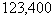(answer/discussion to 1a)Practice Problem 2a: Write the number in decimal notation, without exponents.

 2a.(answer/discussion to 2a)Practice Problems 3a - 3b: Perform the indicated operation. Express the answer in decimal form.

 3a.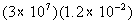(answer/discussion to 3a) 3b.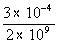(answer/discussion to 3b)Need Extra Help on these Topics?

The following are webpages that can assist you in the topics that were covered on this page:

 http://www.wtamu.edu/academic/anns/mps/math/mathlab/int_algebra/int_alg_tut23_exppart1.htm The bottom of this web page goes over scientific notation.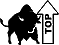WTAMU > Virtual Math Lab > College Algebra

Videos at this site were created and produced by Kim Seward and Virginia Williams Trice.
Last revised on Dec. 4, 2009 by Kim Seward.